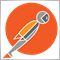# Function to Calculate Number of Opened Symbols168

Could anybody please assist me as I don't know where I got this code wrong.
I am trying to create a  function named "OrdersTotalSymbolOpen()"  to check for open trade on any of the following pairs namely EURUSD, GBPUSD, USDJPY, EURJPY and GBPJPY. It then assigns value 1 to such pair otherwise the value will be equal 0.The function is to return the value of variable "x" which is the sum of  the number of pairs opened out of the 5 pairs.

The problem is that it keeps returning value "0" even when the 5 pairs are opened.

```string       strSymbol;
int x = 0;
int a = 0;
int b = 0;
int c = 0;
int d = 0;
int e = 0;

int init() {
return (0);
}

int deinit() {
return (0);
}

int start() {
Alert ("Total = " + x);
}

int OrdersTotalSymbolOpen() {

a = 0;
b = 0;
c = 0;
d = 0;
e = 0;
strSymbol = OrderSymbol();

for(int i =0;i<OrdersTotal();i++)

{

if (!OrderSelect(i,SELECT_BY_POS)) continue;
if (OrderType() == OP_SELL || OrderType() == OP_BUY)
if (strSymbol=="EURUSD")

a++;
if(strSymbol =="GBPUSD")
b++;
if (strSymbol=="USDJPY")
c++;
if (strSymbol =="EURJPY")
d++;
if (strSymbol=="GBPJPY")
e++;
}
return a;
return b;
return c;
return d;
return e;
if (a>0) {a=1;}else {a=0;}
if (b>0) {b=1;} else {b=0;}
if (c>0) {c=1;} else {c=0;}
if (d>0) {d=1;}else {d=0;}
if (e>0) {e=1;}else {e=0;}

x = a+b+c+d+e;

return x;

}
```Moderator
20380

Olufemi Odunuga:
Could anybody please assist me as I don't know where I got this code wrong.
I am trying to create a  function named "OrdersTotalSymbolOpen()"  to check for open trade on any of the following pairs namely EURUSD, GBPUSD, USDJPY, EURJPY and GBPJPY. It then assigns value 1 to such pair otherwise the value will be equal 0.The function is to return the value of variable "x" which is the sum of  the number of pairs opened out of the 5 pairs.

The problem is that it keeps returning value "0" even when the 5 pairs are opened.

To be honest with you, I cannot even be bothered to read your code.

Why?

Because you do not use meaningful names for the variables.

Get into the habit of using variable names to describe what they are storing. Especially if you want others to help you.168

Thanks Keith,

Your observation is noted. I was just trying to make it simple. I thought my explanation would suffice.Moderator
20380

Olufemi Odunuga:

Thanks Keith,

Your observation is noted. I was just trying to make it simple. I thought my explanation would suffice.

It doesn't make it simple....

```if(NumberOfTrades<MaxTrades)
{
//open the order
}```

is much easier to understand than

```if(N<M)
{
//open the order
}```

as you don't have to check back through the code to find out what values N and M hold.2392

Olufemi Odunuga:

Thanks Keith,

Your observation is noted. I was just trying to make it simple. I thought my explanation would suffice.

It's not simple, it's spaghetti code. You need to create a collection of unique elements, and in programming the normal way to achieve this is by using a set. Unfortunately, MQL doesn't have a "set" collection for strings but you can easily subclass CArrayString and make your own.

...actually I've done it for you...

```#include <Arrays\ArrayString.mqh>
class StringSet : public CArrayString
{
if(this.SearchLinear(element) < 0)
return false;
}
};

void OnStart()
{
StringSet unique_symbols;
for(int i=OrdersTotal()-1; i>=0; --i)
if(OrderSelect(i, SELECT_BY_POS) && OrderType() < 2)